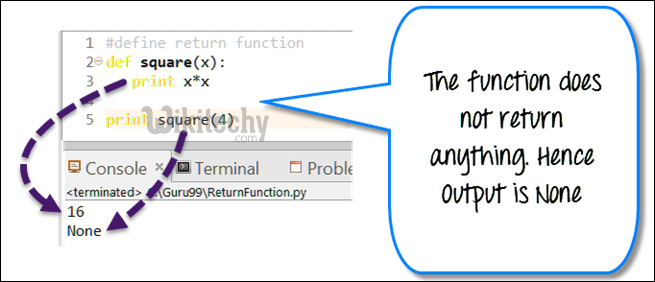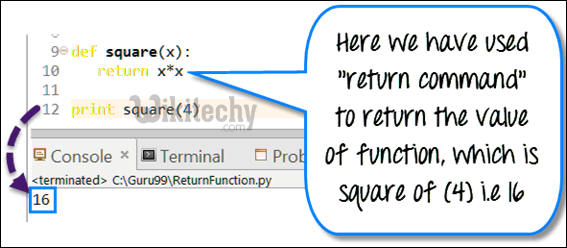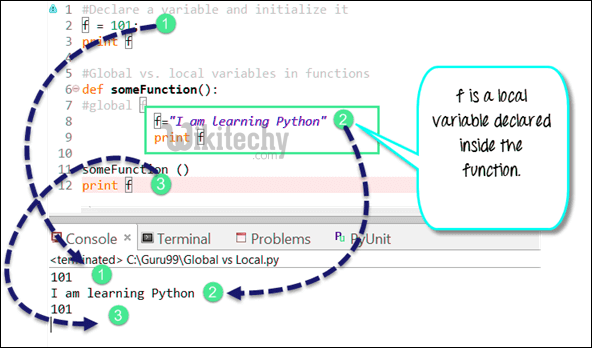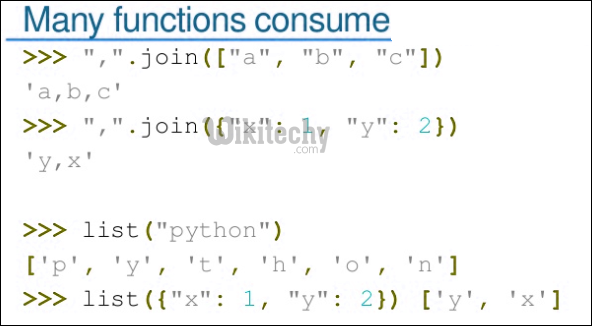# python tutorial - Python Pass | How to write an empty function in Python – pass statement - learn python - python programmingLearn Python - Python tutorial - Pass Arguments Python - Python examples - Python programs

In C/C++ and Java, we can write empty function as followingpython - Sample - python code :

```// An empty function in C/C++/Java
void fun() {  }
```

In Python, if we write something like following in Python, it would produce compiler error.python - Sample - python code :

python tutorial - Output :

`IndentationError: expected an indented block`

In Python, to write empty functions, we use pass statement. pass is a special statement in Python that does nothing. It only works as a dummy statement.python - Sample - python code :

We can use pass in empty while statement also.python - Sample - python code :

We can use pass in empty if else statements.python - Sample - python code :

Wikitechy tutorial site provides you all the learn python , python course online free , free python training , python programming learning , free python course